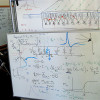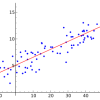# Archive | R Machine Learning## Non-Linear Classification in R

In this post you will discover 8 recipes for non-linear classification in R. Each recipe is ready for you to copy and paste and modify for your own problem. All recipes in this post use the iris flowers dataset provided with R in the datasets package. The dataset describes the measurements if iris flowers and requires classification of […]## Linear Classification in R

In this post you will discover recipes for 3 linear classification algorithms in R. All recipes in this post use the iris flowers dataset provided with R in the datasets package. The dataset describes the measurements if iris flowers and requires classification of each observation to one of three flower species. Let’s get started. Logistic […]## Non-Linear Regression in R with Decision Trees

In this post, you will discover 8 recipes for non-linear regression with decision trees in R. Each example in this post uses the longley dataset provided in the datasets package that comes with R. The longley dataset describes 7 economic variables observed from 1947 to 1962 used to predict the number of people employed yearly. Let’s get started. Classification […]## Non-Linear Regression in R

In this post you will discover 4 recipes for non-linear regression in R. There are many advanced methods you can use for non-linear regression, and these recipes are but a sample of the methods you could use. Let’s get started. Each example in this post uses the longley dataset provided in the datasets package that comes with R. The […]## Penalized Regression in R

In this post you will discover 3 recipes for penalized regression for the R platform. You can copy and paste the recipes in this post to make a jump-start on your own problem or to learn and practice with linear regression in R. Let’s get started. Each example in this post uses the longley dataset provided in the datasets […]## Linear Regression in R

In this post you will discover 4 recipes for linear regression for the R platform. You can copy and paste the recipes in this post to make a jump-start on your own problem or to learn and practice with linear regression in R. Let’s get started. Each example in this post uses the longley dataset […]## Applied Machine Learning Lessons from A Case Study of Passenger Survival Prediction

A valuable exercise when learning and practicing machine learning is to study how others apply methods and solve problems. It’s valuable because you can learn about new processes, software, graphs, and algorithms. But it is new ways of thinking about the process of solving problems with machine learning that is the most valuable part of […]## Books for Machine Learning with R

R is a powerful platform for data analysis and machine learning. It is my main workhorse for things like competitions and consulting work. The reason is the large amounts of powerful algorithms available, all on the one platform. In this post I want to point out some resources you can use to get started in […]